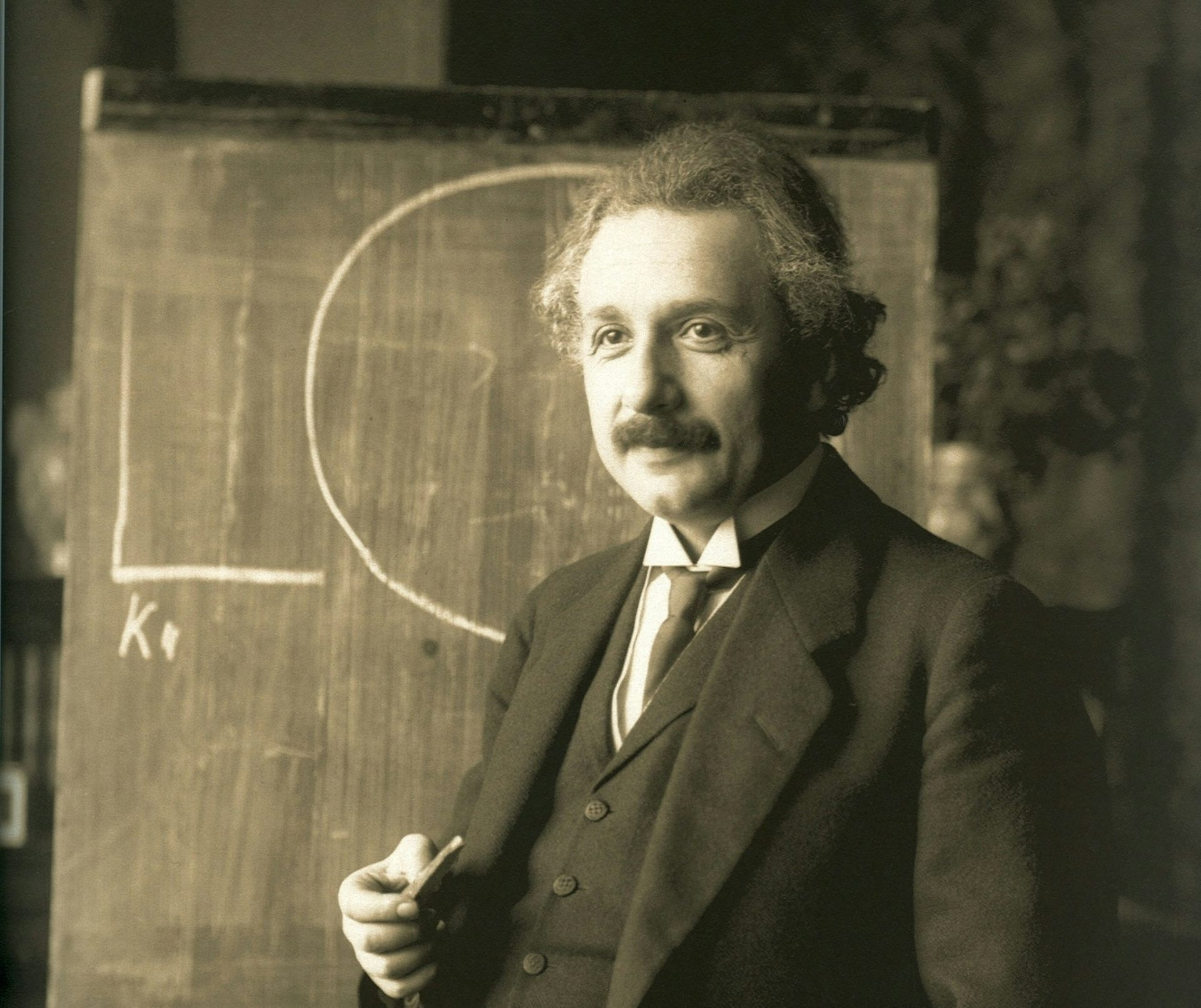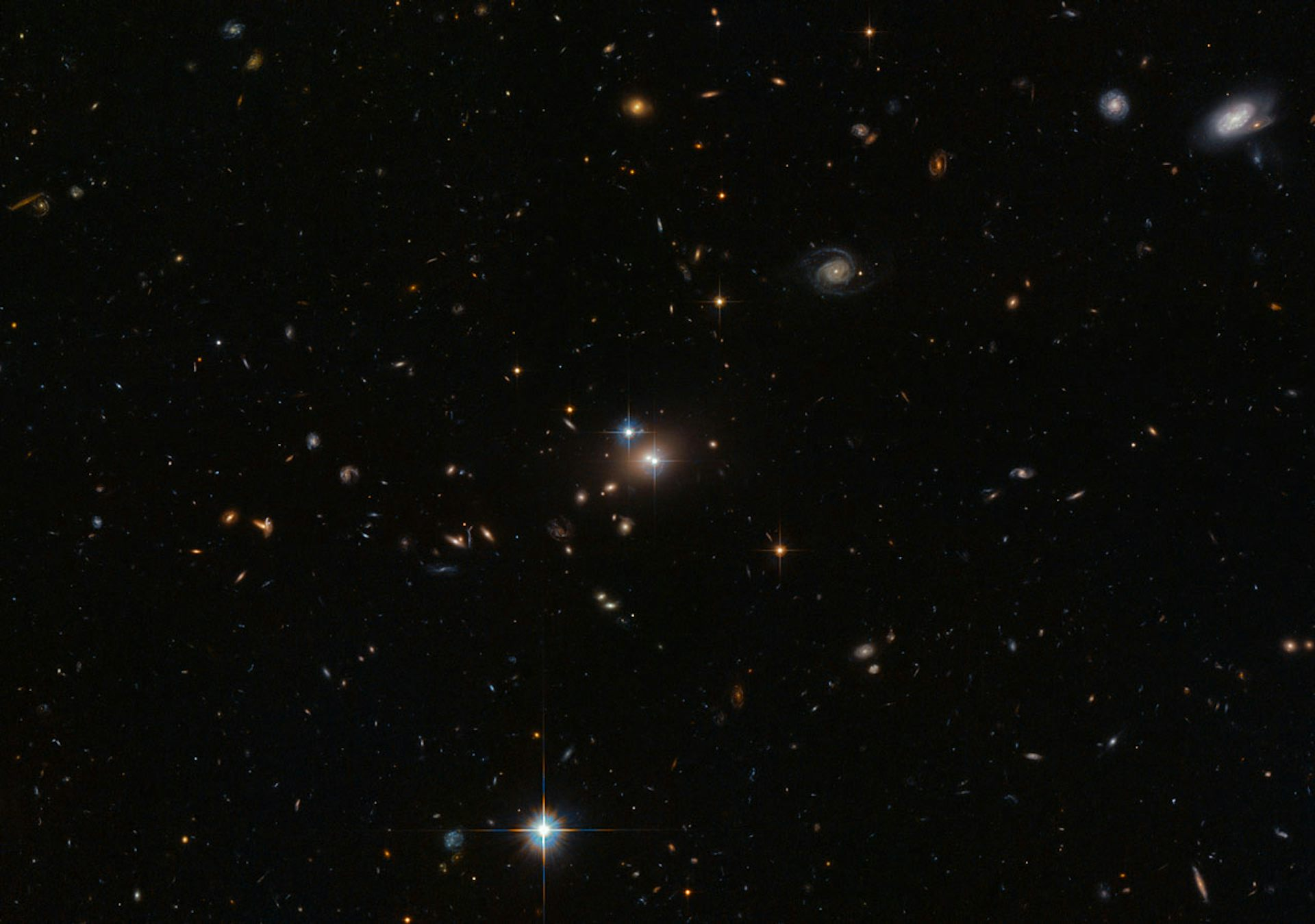It’s possible that had Einstein not conceived of general relativity, then we’d still be at a loss to explain gravity to this day. Wikimedia

It was 1905, and Albert Einstein had just turned theoretical physics on its head by publishing a paper on what later became known as special relativity. This showed that space and time could not be considered in absolute terms: time could speed up or slow down; standard lengths could contract; and masses could increase.

And, most famously, energy was equivalent to mass, proportional to each other based on the famous equation E = mc².

Although there is no doubting Einstein’s genius in formulating special relativity, it is generally accepted that had Einstein not published the theory in 1905, some other physicist would have done so shortly thereafter.The ‘Einstein cross’ is actually four images of the same distant quasar that have been bent around a closer galaxy which acts like a gravitational lens. NASA, ESA, and STScI

It was not until 1915 that Einstein’s unparalleled genius was demonstrated when he published his general theory of relativity. This theory made the claim that space–time curvature is proportional to, and is caused by, the “energy-momentum density”, that is, the energy and the momentum associated with all and any kind of matter in a unit volume of space.

This claim was subsequently confirmed when it was shown to fit with observations of Mercury’s unusual orbit and the bending of starlight around the sun.

And over the course of the last century, general relativity has been tested to remarkable accuracy and has withstood critique each time. General relativity was such a giant leap forward that it is arguable that had Einstein not formulated the theory, it may have remained undiscovered for a very long time.

Path to general relativity

In 1907, Einstein had the “happiest thought of my life” when he was sitting in a chair at the patent office in Bern:

If a person falls freely he will not feel his own weight.

This led him to the postulate the “equivalence principle”, which states that one cannot tell the difference between an accelerating reference frame and a gravitational field. For example, standing on the Earth feels the same as standing in a space craft accelerating at 9.81 meters per second per second, which is the acceleration due to gravity on the Earth’s surface.

This was the crucial first step in Einstein’s formulation of a new theory of gravity.

Next, Einstein believed that, “all physics is geometry”. By that he meant we can think of space-time and all the universe in terms of geometry. The most startling conclusion from special relativity – the dynamic nature of time and space – must have led Einstein to start considering “geometric” space-time to be in need of modification.

Einstein then embarked on a series of careful thought experiments, comparing observations made by observers in inertial and rotating frames.

Einstein deduced that for observers in a rotating frame, space time could not be Euclidean, i.e. it could not be like the kind of flat geometry we all learn about in school. Rather, we need to introduce “curved space” to account for the anomalies predicted by relativity. Curvature thus became the second key assumption that underpinned his general theory.

To describe curved space-time, Einstein called upon the earlier work of Bernhard Riemann, a 19th century mathematician. And with the help of his friend Marcel Grossman, also a mathematician, Einstein spent several exhausting years learning the mathematics of curved spaces, or what mathematicians call “differential geometry”. As Einstein commented, “compared with understanding gravity, special relativity was mere child’s play”.

Now Einstein had the mathematical tools to finalise his theory. His equivalence principle stated that an accelerating reference frame is equivalent to a gravitational field. From his geometrical studies, he believed the gravitational field was simply a manifestation of curved space-time. Hence, Einstein could show that accelerating frames were represented by non-Euclidean space.

It follows

The third key step for Einstein involved resolving complications that had arisen when special relativity was applied to Newtonian gravitational physics. In special relativity, the constancy of the speed of light in all reference frames, and the conclusion that the speed of light set the universal speed limit, was in direct contradiction to Newton’s theory of gravity, which postulated the instantaneous effect of gravity.

Put simply: Newtonian gravity predicted that if the sun was removed from the centre of the solar system, the gravitational effect on the Earth would be instantaneous. However, special relativity says that even the gravitational effect of the sun disappearing ought to travel at the speed of light.

Einstein also knew that gravitational force between two bodies was directly proportional to their masses, from Newton’s equation F = GMm/r². So mass clearly determined the strength of the gravitational field. Special relativity tells us that mass is equivalent to energy, so the energy–momentum density must also determine the gravitational force.

Thus the three key assumptions that Einstein used to formulate his theory were:

1. Rotating frames (non-inertial) imply non-Euclidean (or curved) space-time

2. The equivalence principle asserts that accelerating frames (i.e. non inertial frames) are equivalent to gravitational fields

3. From special relativity, mass is equal to energy, and from Newtonian physics, mass is proportional to strength of gravity.

Hence, Einstein was able to conclude that energy-momentum density causes, and is proportional to, space-time curvature.

It is not clear when Einstein had the “light bulb moment”, the moment when he was able to put the puzzle together and link mass/energy with the curvature of space.

From 1913 to 1915, Einstein published several papers as he laboured to complete the general theory. Some of these papers contained errors and took Einstein down theoretical pathways that ultimately were not productive.

But the final result, that the energy–momentum density of matter curves space-time, like a bowling ball curves a flat sheet of rubber, and that the motion of a mass in a gravitational field is based on the curvature of space time, just as a bowling ball moves freely on a curved rubber sheet, is surely one of the greatest insights of human intellect.

How long would it have taken us to understand gravity without Einstein’s sheer genius? It is possible we would have had to wait many decades.

However, in 1979 the cat would have been out of the bag. In that year, astronomers discovered the “twin quasar”, QSO 0957+561, the first gravitationally-lensed quasar.The discovery of the ‘twin quasar’ would likely have tipped off physicists to the curvature of space-time had Einstein not beat them to it. NASA/ESA

This astonishing discovery could only have made sense if space-time was curved. It would surely have attracted a Nobel Prize if it hadn’t been for Einstein’s genius. Maybe it still should.

Want to write?

Write an article and join a growing community of more than 91,000 academics and researchers from 2,981 institutions.